# Selina Solutions Concise Maths Class 10 Chapter 22 Heights and Distances Exercise 22(B)

Different models of problems are included in this exercise to provide a deeper understanding of the concepts of finding heights and distances. The Selina Solutions for Class 10 Maths is the right resource for students to clarify their doubts instantly, by identifying the right procedures to solve problems. The solutions to the Concise Selina Solutions for Class 10 Maths Chapter 22 Heights and Distances Exercise 22(B) are available in PDF format, provided in the link given below.

## Concise Selina Solutions for Class 10 Maths Chapter 22 Heights and Distances Exercise 22(B) Download PDF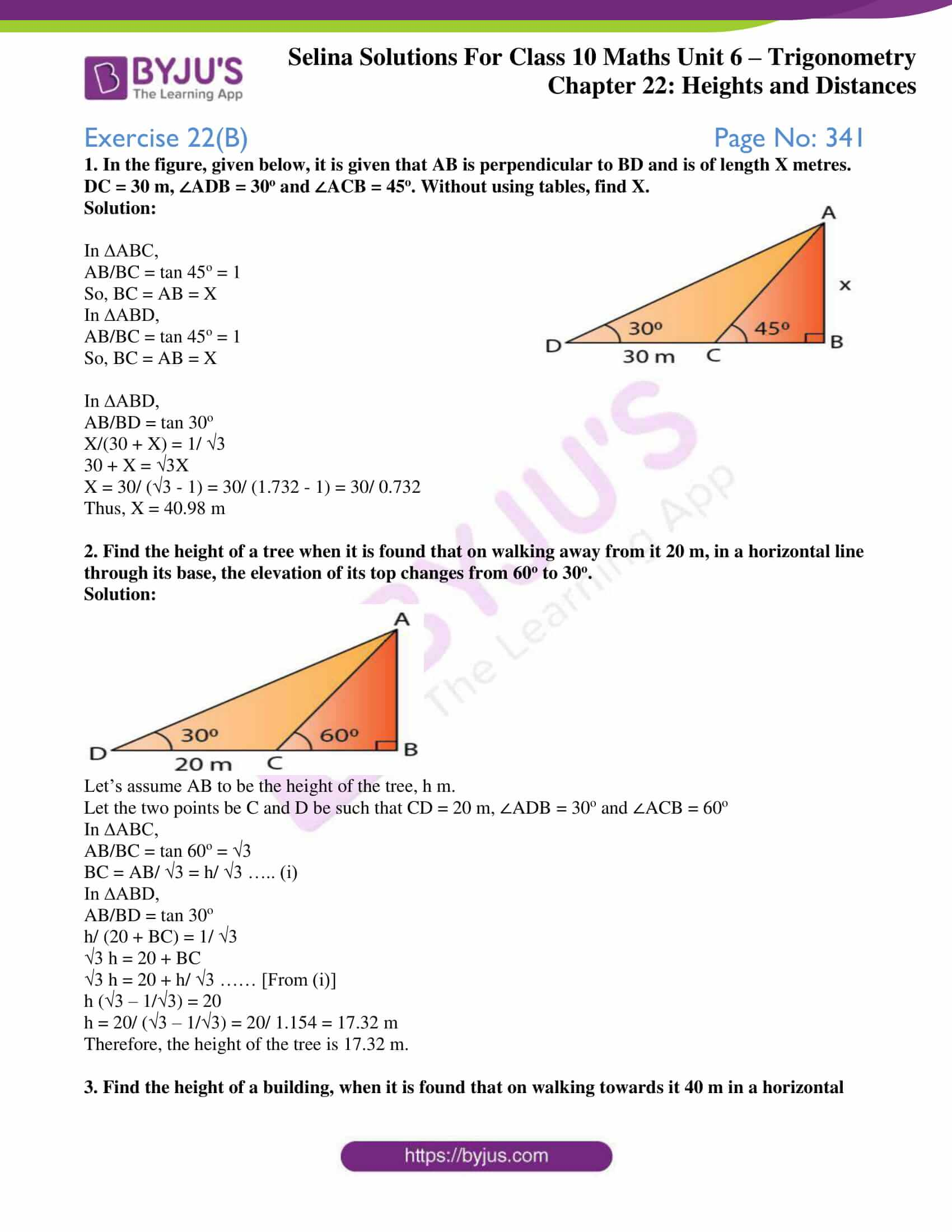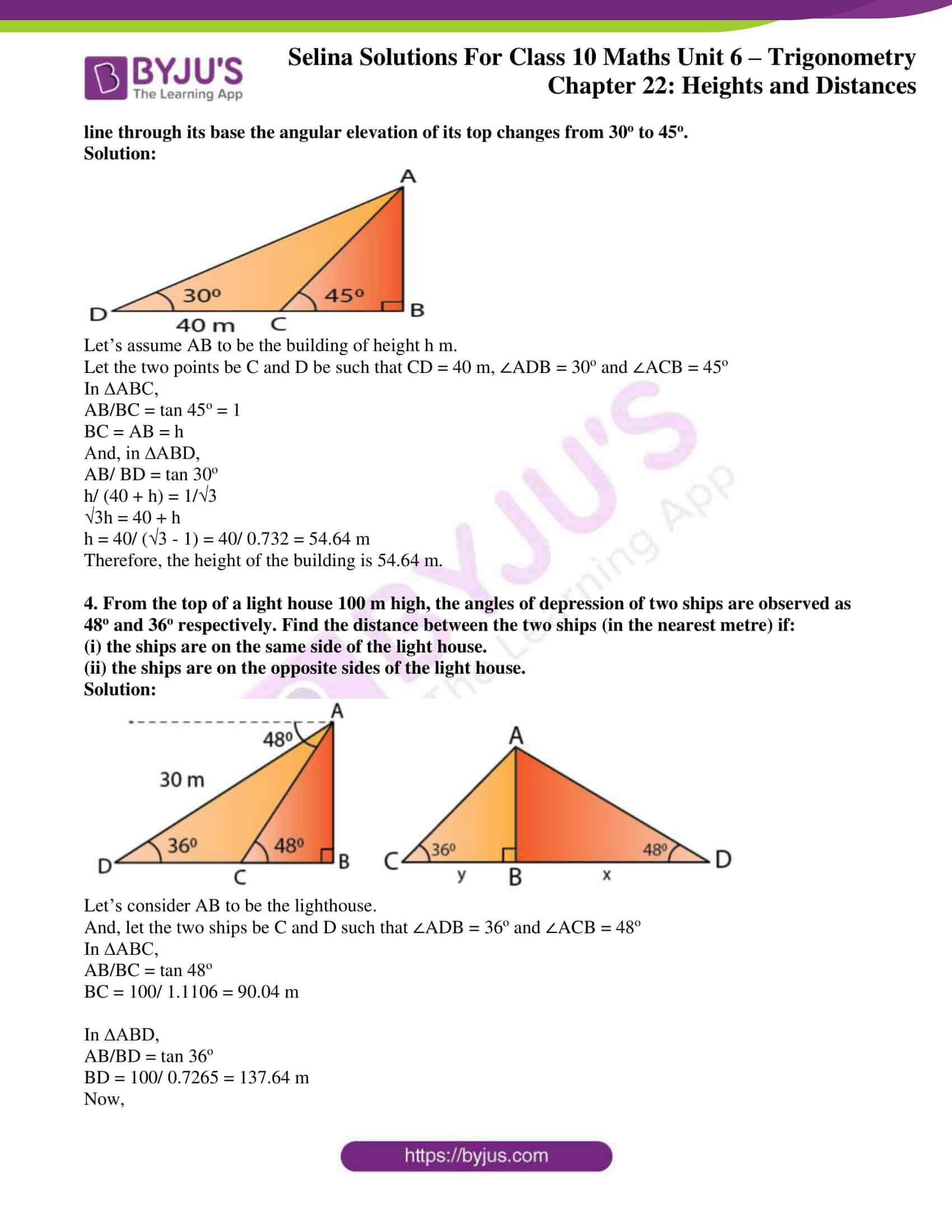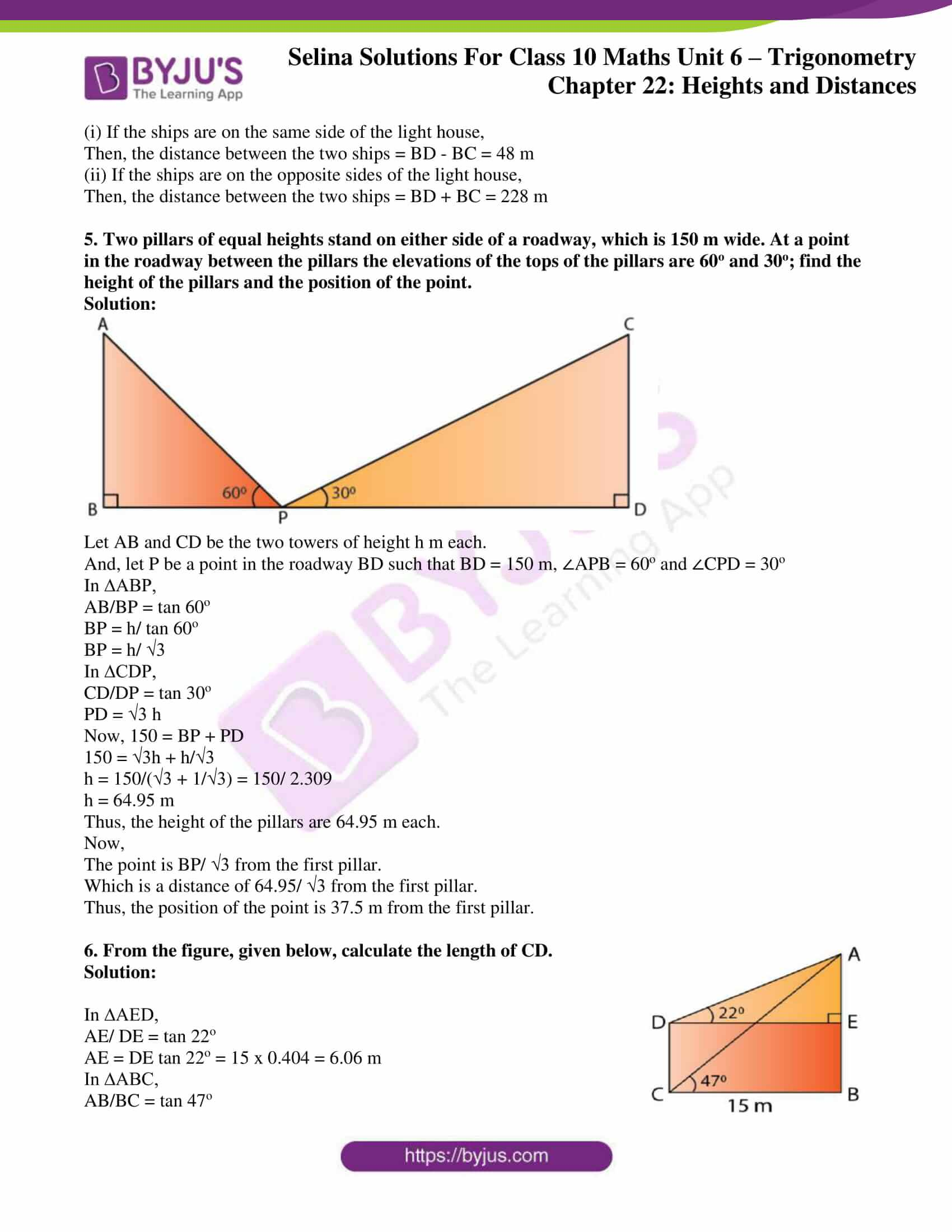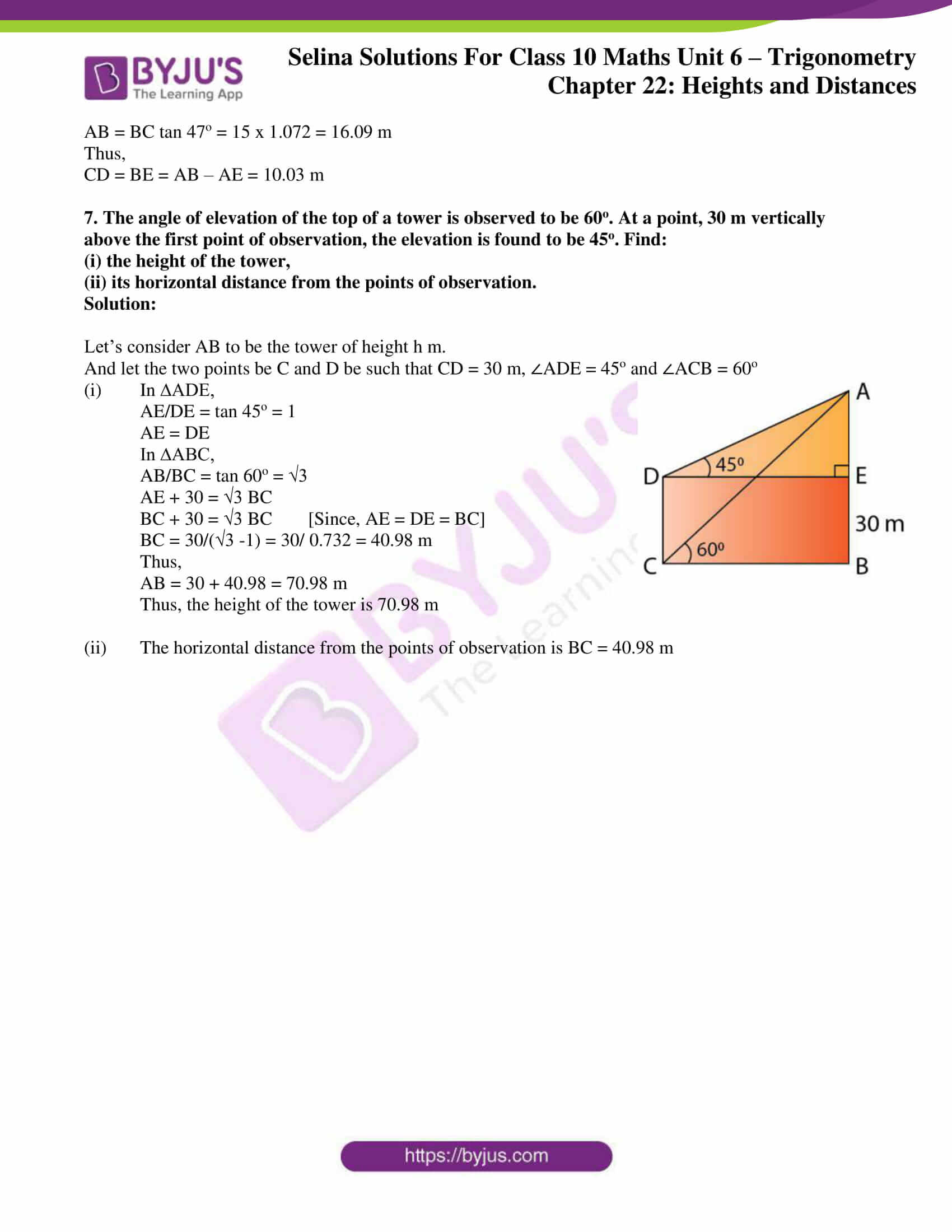### Access other exercises of Selina Solutions Concise Maths Class 10 Chapter 22 Heights and Distances

Exercise 22(A) Solutions

Exercise 22(C) Solutions

### Access Selina Solutions Concise Maths Class 10 Chapter 22 Heights and Distances Exercise 22(B)

1. In the figure, given below, it is given that AB is perpendicular to BD and is of length X metres. DC = 30 m, ∠ADB = 30o and ∠ACB = 45o. Without using tables, find X.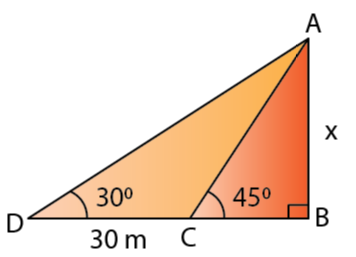Solution:

In ∆ABC,

AB/BC = tan 45o = 1

So, BC = AB = X

In ∆ABD,

AB/BC = tan 45o = 1

So, BC = AB = X

In ∆ABD,

AB/BD = tan 30o

X/(30 + X) = 1/ √3

30 + X = √3X

X = 30/ (√3 – 1) = 30/ (1.732 – 1) = 30/ 0.732

Thus, X = 40.98 m

2. Find the height of a tree when it is found that on walking away from it 20 m, in a horizontal line through its base, the elevation of its top changes from 60o to 30o.

Solution: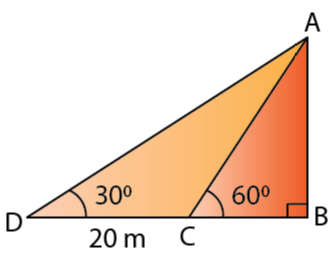Let’s assume AB to be the height of the tree, h m.

Let the two points be C and D be such that CD = 20 m, ∠ADB = 30o and ∠ACB = 60o

In ∆ABC,

AB/BC = tan 60o = √3

BC = AB/ √3 = h/ √3 ….. (i)

In ∆ABD,

AB/BD = tan 30o

h/ (20 + BC) = 1/ √3

√3 h = 20 + BC

√3 h = 20 + h/ √3 …… [From (i)]

h (√3 – 1/√3) = 20

h = 20/ (√3 – 1/√3) = 20/ 1.154 = 17.32 m

Therefore, the height of the tree is 17.32 m.

3. Find the height of a building, when it is found that on walking towards it 40 m in a horizontal line through its base the angular elevation of its top changes from 30o to 45o.

Solution: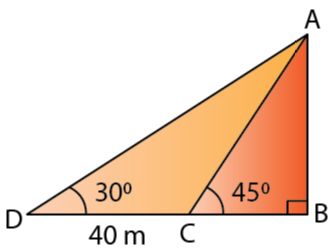Let’s assume AB to be the building of height h m.

Let the two points be C and D be such that CD = 40 m, ∠ADB = 30o and ∠ACB = 45o

In ∆ABC,

AB/BC = tan 45o = 1

BC = AB = h

And, in ∆ABD,

AB/ BD = tan 30o

h/ (40 + h) = 1/√3

√3h = 40 + h

h = 40/ (√3 – 1) = 40/ 0.732 = 54.64 m

Therefore, the height of the building is 54.64 m.

4. From the top of a light house 100 m high, the angles of depression of two ships are observed as 48o and 36o respectively. Find the distance between the two ships (in the nearest metre) if:

(i) the ships are on the same side of the light house.

(ii) the ships are on the opposite sides of the light house.

Solution: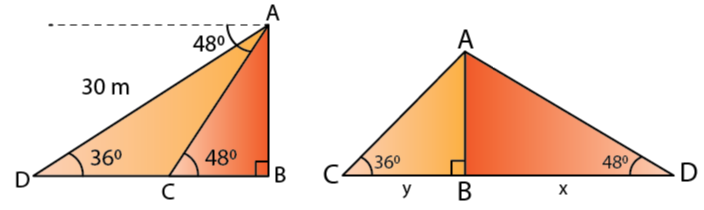Let’s consider AB to be the lighthouse.

And, let the two ships be C and D such that ∠ADB = 36o and ∠ACB = 48o

In ∆ABC,

AB/BC = tan 48o

BC = 100/ 1.1106 = 90.04 m

In ∆ABD,

AB/BD = tan 36o

BD = 100/ 0.7265 = 137.64 m

Now,

(i) If the ships are on the same side of the light house,

Then, the distance between the two ships = BD – BC = 48 m

(ii) If the ships are on the opposite sides of the light house,

Then, the distance between the two ships = BD + BC = 228 m

5. Two pillars of equal heights stand on either side of a roadway, which is 150 m wide. At a point in the roadway between the pillars the elevations of the tops of the pillars are 60o and 30o; find the height of the pillars and the position of the point.

Solution: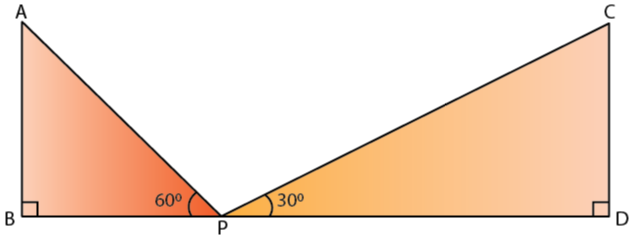Let AB and CD be the two towers of height h m each.

And, let P be a point in the roadway BD such that BD = 150 m, ∠APB = 60o and ∠CPD = 30o

In ∆ABP,

AB/BP = tan 60o

BP = h/ tan 60o

BP = h/ √3

In ∆CDP,

CD/DP = tan 30o

PD = √3 h

Now, 150 = BP + PD

150 = √3h + h/√3

h = 150/(√3 + 1/√3) = 150/ 2.309

h = 64.95 m

Thus, the height of the pillars are 64.95 m each.

Now,

The point is BP/ √3 from the first pillar.

Which is a distance of 64.95/ √3 from the first pillar.

Thus, the position of the point is 37.5 m from the first pillar.

6. From the figure, given below, calculate the length of CD.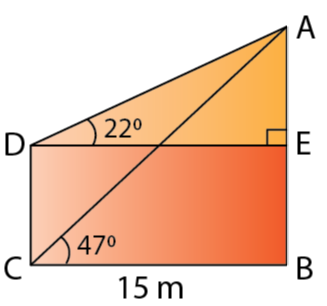Solution:

In ∆AED,

AE/ DE = tan 22o

AE = DE tan 22o = 15 x 0.404 = 6.06 m

In ∆ABC,

AB/BC = tan 47o

AB = BC tan 47o = 15 x 1.072 = 16.09 m

Thus,

CD = BE = AB – AE = 10.03 m

7. The angle of elevation of the top of a tower is observed to be 60o. At a point, 30 m vertically above the first point of observation, the elevation is found to be 45o. Find:

(i) the height of the tower,

(ii) its horizontal distance from the points of observation.

Solution:

Let’s consider AB to be the tower of height h m.

And let the two points be C and D be such that CD = 30 m, ∠ADE = 45o and ∠ACB = 60o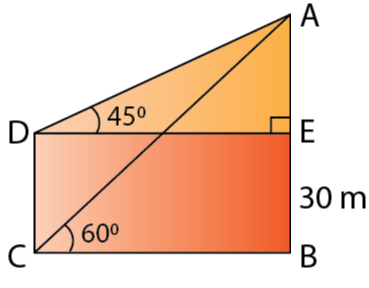(i) In ∆ADE,

AE/DE = tan 45o = 1

AE = DE

In ∆ABC,

AB/BC = tan 60o = √3

AE + 30 = √3 BC

BC + 30 = √3 BC [Since, AE = DE = BC]

BC = 30/(√3 -1) = 30/ 0.732 = 40.98 m

Thus,

AB = 30 + 40.98 = 70.98 m

Thus, the height of the tower is 70.98 m

(ii) The horizontal distance from the points of observation is BC = 40.98 m